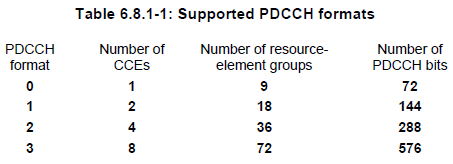LTE Quick Reference PDCCH Resource Allocation   One PDCCH is carried by multiple number of consecutive CCEs. How many CCEs are necessary to carry one PDCCH ? It depends on the format of the PDCCH. The relationship between PDCCH format and the number CCE required to carry the PDCCH is as follows : PDCCH Format 0 : Requires 1 CCE = Aggregation Level 1 (2^PDCCH Format = 2^0 = 1) PDCCH Format 1 : Requires 2 CCE = Aggregation Level 2 (2^PDCCH Format = 2^1 = 2) PDCCH Format 2 : Requires 4 CCE = Aggregation Level 4 (2^PDCCH Format = 2^2 = 4) PDCCH Format 3 : Requires 8 CCE = Aggregation Level 8 (2^PDCCH Format = 2^3 = 8)   The number of consecutive CCEs required to carry one PDCCH is called "Aggregation Level'. TS 36.211 Table 6.8.1.1 shows these relations.Now let's look into a little bit deeper and see how 'Number of PDCCH bits' is derived for each PDCCH   Number of PDCCH bits for PDCCH Format 0 = 1 (CCE) x 9 (REG/CCE) x 4 (RE/REG) x 2 (bits/RE, QPSK) = 72 Number of PDCCH bits for PDCCH Format 1 = 2 (CCE) x 9 (REG/CCE) x 4 (RE/REG) x 2 (bits/RE, QPSK) = 144 Number of PDCCH bits for PDCCH Format 2 = 4 (CCE) x 9 (REG/CCE) x 4 (RE/REG) x 2 (bits/RE, QPSK) = 288 Number of PDCCH bits for PDCCH Format 3 = 8 (CCE) x 9 (REG/CCE) x 4 (RE/REG) x 2 (bits/RE, QPSK) = 576   What does this mean in practical sense ? It means that even the same DCI with exactly same bit length, the number of physical channel bits gets different depending on which PDCCH format it is carried by. It means the Code Rate of PDCCH varies depending on which PDCCH format is used.   For example, if we use DCI Format 2A (I don’t remember the exact bit length… but it would be around 40 bits) Case 1 : If we use Aggregation Level = 1 i)  After Channel Coding, the bit length would be about 120 ii) After Rate Matching, it should be 72 (1 CCE)   Case 2 : If we use Aggregation Level = 2 i)  After Channel Coding, the bit length would be about 120 ii) After Rate Matching, it should be 144 (2 CCE)   Case 3 : If we use Aggregation Level = 4 i)  After Channel Coding, the bit length would be about 120 ii) After Rate Matching, it should be 288 (4 CCE)   Case 4 : If we use Aggregation Level = 8 i)  After Channel Coding, the bit length would be about 120 ii) After Rate Matching, it should be 576 (8 CCE)